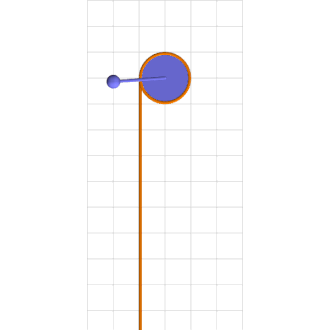# Simplest Chaos

The motion of one of the simplest dynamical systems, a torqued, damped, nonlinear pendulum, can be infinitely complicated.

Consider a simple pendulum of length $l$ and mass $m$ rigidly connected to an axle of radius $r$ wrapped by a rope that hangs down one side with a mass $M$ climbing up and down it, as in the attached animation.

If the climber’s height varies sinusoidally (relative to the axle)

$$x = x_0 + \frac{a}{\omega^2} \sin \omega t,$$

then its acceleration also varies sinusoidally

$$\ddot x = - a \sin \omega t,$$

so the total force on the climber

$$M\ddot x = \sum_\text{down} f = Mg-T$$

implies upper rope tension

$$T = Mg + ma \sin \omega t,$$

where $0 < a < g$. If the axle and rope have negligible inertia, then the total torque on the axle

$$m l^2 \ddot \theta = \sum_\text{CCW}\tau = - mgl \sin\theta + rT - \gamma \dot\theta,$$

where $\gamma$ is the axle viscosity. The full motion equation

$$m l^2 \ddot \theta = - mgl \sin\theta + rMg + rma \sin \omega t - \gamma \dot\theta$$

reduces to

$$\ddot \theta = - \sin\theta + 0.7155 + 0.4 \sin 0.25 t - 0.75 \dot\theta$$

for parameters that describe the animation chaotic motion.Green mass sinusoidally climbs up and down brown rope torquing blue pendulum into chaotic motion.
Upper rope tension is largest with climber lowest and smallest with climber highest.